Courses
Courses for Kids
Free study material
Free LIVE classes
More

# Congruence of Plane FiguresLIVE
Join Vedantu’s FREE Mastercalss

## Introduction

Two shapes are said to be congruent if they are equal to each other in all possible ways. In Mathematics, congruent figures are those figures which have the same shape and the same size. The congruence applies to both the 2-D and 3-D figures. But in this article, we shall discuss the congruence of plane figures only.

Congruent figures are those having the same shape and size. The relation used in the two congruent figures is known as congruence. It is denoted by the symbol $\cong$.

## Congruent Figure

In Mathematics, Congruent figures are those geometric figures that have the same shape and size. If you transform one figure into another figure by a sequence of rotations, and/or reflections, then the two figures are equal to each and hence they are called congruent figures. For more illustration, we take two figures and prove them congruent.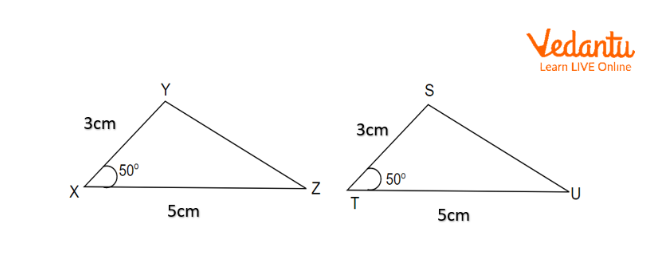Two Congruent Triangles

In $\Delta XYZ$ and $\Delta STU$ here,

$\Rightarrow XY = ST = 3cm$

$\Rightarrow \angle YXZ = \angle STU = 50^\circ$

$\Rightarrow XZ = TU = 5cm$

These two triangles are congruent with each by the property S.A.S (Side-Angle-Side).

Therefore, $\Delta XYZ \cong \Delta STU$.

## Congruence of Plane Figures

A plane figure is a geometric figure consisting of no thickness. Line segments, curves or a combination of both line segments and curves are some of the plane figures. The straight or curved lines that make up the plane figures are called their sides.

Plane figures such as line segments, angles and other figures are said to be congruent if they have the same shape and size. The relation used is known as the congruence of plane figures. For example,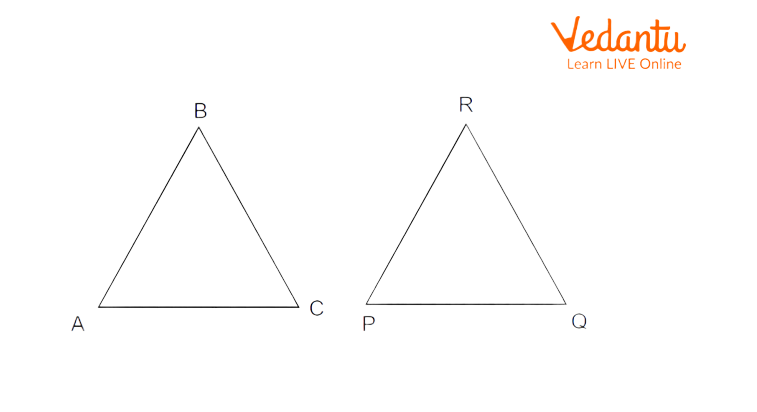Two Congruent Figures

Here, these planes are congruent with each other because both of these figures have the same shape and size.

## Congruent Segments Definition

The congruent segment is a set of two line segments that have equal lengths. A line segment is a straight line with specific starting and ending points. As it has specific starting and ending points, its length can be measured. Congruent line segments don’t need to be parallel, perpendicular or at any specific angle to each other, but they can be.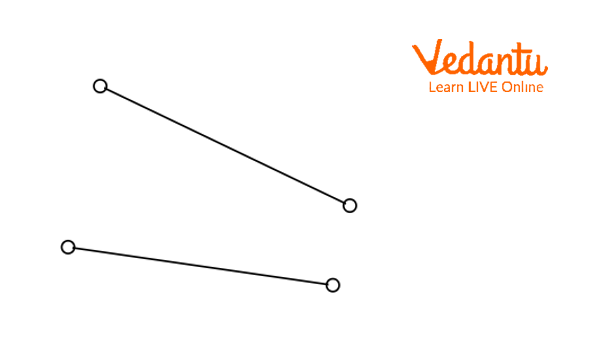Two Congruent Line Segments

## Congruence of Line Segment

A line segment is a primary figure in the geometry that is formed by connecting any two points of the plane figure. The sides of the plane figures are also line segments. If two line segments have the same length, then they are said to be congruent. In other words, if two line segments are congruent, then their lengths are equal.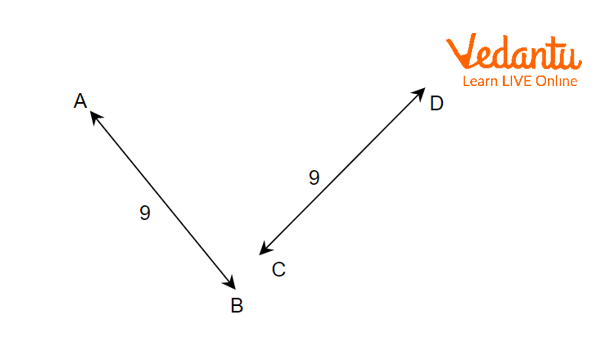Two Line Segments

In the above figures, two line segments $\overline {AB}$ and $\overline {CD}$ have equal lengths of 9 units. Two line segments $\overline {AB}$ and $\overline {CD}$ superimpose on each other. So, they are congruent with each other. Hence, the line segments $\overline {AB}$ and $\overline {CD}$ are congruent lines.

$\overline {AB} \cong \overline {CD}$

## Two Line Segments are Congruent

Two line segments are congruent if they have the same length. However, they don't need to be parallel. They can be oriented at any angle or can be at any position. A line segment is defined by the distance between two points.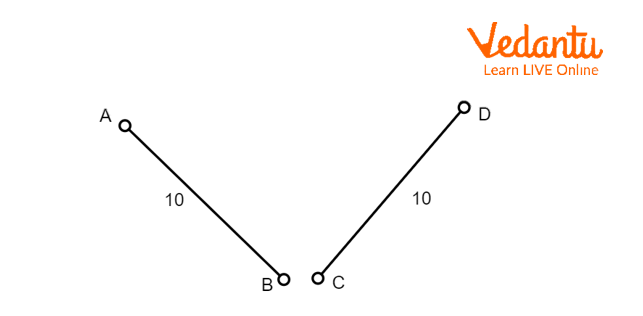Two Congruent Line Segments

In the figures given above, the line segment has the same length that's why line AB is congruent with line CD. This statement can also be written as $AB \cong CD$.

## Plane Shapes Images

A closed 2-D or flat figure is called a plane shape. Different plane shapes have different properties, such as the number of vertices. A side is a straight line that is included in the shape, and a vertex is where two sides meet each other. Some of the basic plane shapes are triangles, squares, rectangles, ovals, circles and polygons whose figures are given below.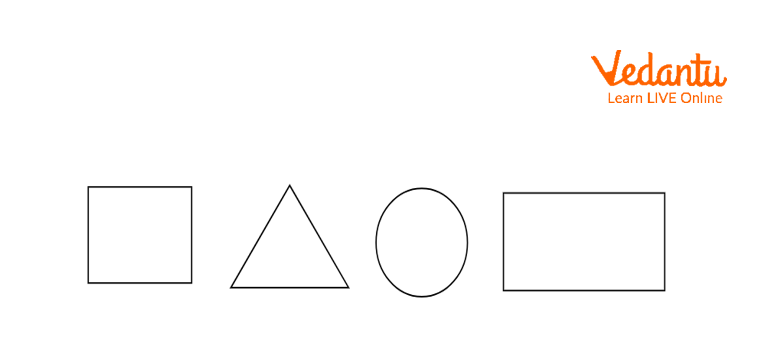Plane Figures

### Interesting Facts

• The other name for the plane figure is a paraboloid.

• Gottfried Wilhelm Leibniz is the father of congruence.

• The hexagon is the strongest plane shape known.

### Solved Examples Related to Congruence of Plane Figures

1. If two triangles ABC and PQR are congruent, then find $\angle PQR$.Solution: Given that the $\Delta ABC$ and $\Delta PQR$ are congruent to each other.

$\angle BAC = \angle QPR = 40^{\circ}$, $\angle ACB = \angle PRQ = 60^{\circ}$, and $\angle CBA = \angle RQP$.

The sum of all angles of a triangle is $180^{\circ}$.

$\angle QPR + \angle PRQ + \angle RQP = 180^{\circ}$

$\Rightarrow 40^{\circ} + 40^{\circ}+ \angle RQP = 180^{\circ}$

$\Rightarrow 100^{\circ} + \angle RQP = 180^{\circ}$

$\Rightarrow \angle RQP = 80^{\circ}$

Therefore $\angle RQP = 80^{\circ}$.

2. If two shapes are congruent to each other and the perimetre of the first figure is 180 m, then find the perimeter of another shape.

Solution: Since both shapes are congruent to each other, the perimeter of both shapes will be the same. The perimeter of another shape is 180 cm.

### Key Features

• Two figures are congruent if they possess the same shape and size, or if the figure has the same shape and size as its mirror image, then it is congruent to its mirror image.

• Plane figures are those figures which are drawn on a plane or 2-D surface.

• A plane in geometry is a flat surface that can extend up to infinity in all directions. As it is extended to infinity, it has infinite width and length, has no thickness and has curvature.

### Practice Questions

If two regular pentagons are congruent and the perimeter of one of them is 30 cm, then find the length of one side of another pentagon.

Last updated date: 26th Sep 2023
Total views: 91.2k
Views today: 2.91k

## FAQs on Congruence of Plane Figures

1. What is the difference between congruent and similar shapes?

The similar shape is a resized version of the original shape. The congruent shapes are identical to each other.

2. What are the conditions of two triangles that are congruent?

The conditions are:

• SSS (side-side-side)

• SAS (side-angle-side)

• AAA (angle-angle-angle)

• ASA (angle-side-angle)

• RHS (Right angle-hypotenuse-side)

3. Why are congruent shapes always similar?

In similar shapes, the size of the shapes may not be equal. The ratio of the parameters is always constant for similar shapes. But two shapes are congruent if the sizes of the shapes are the same. Thus congruent shapes are always similar.